Review articles

# Entropy and its significance in transport of ions through the cell membraneS. Pašić*, N. Popara, A. Klobučar, D. Cvitković and M. Vilić

Selim PAŠIĆ*, BSc, PhD, Assistant Professor (Coresponding author, e-mail: selimpasic@gmail.com), Nato POPARA, mag. phys., Assistant, Faculty of Veterinary Medicine, University of Zagreb, Croatia; Antea KLOBUČAR, DVM, Ministry of Defence, Armed Forces of the Republic of Croatia, Croatian Navy, Croatia; Denis CVITKOVIĆ, DVM, PhD, Assistant Professor, Marinko VILIĆ, DVM, PhD, Full Professor, Faculty of Veterinary medicine, University of Zagreb, Croatia

## Abstract

The aim of this paper is to present the concept of entropy in a simple way and to show its key importance in the transport processes of ions through the cell membrane using the latest discoveries in biophysics.
Using a real-life example, we show how processes within a system lead to an increase in entropy. We also show how this entropy increase is directly related to the irreversibility of the process, and how it defines the arrow of time (direction of the flow of time). Using an abstract example, we clarify the meaning of the concept of disorder in a system, which is often used in defining entropy by connecting it with the number of microstates that realise a macroscopic state of a system. The importance of entropy in transport processes of ions through the cell membrane is considered. We show that passive transport processes through the cell membrane are the result of an entropy increase in the cell membrane-transported substance system. A model of active ion transport through the cell membrane following Rubi et al. (2017) is presented. The force that transports ions through the channel in the transport protein arises due to the entropy gradient formed along the transport channel, which is a consequence of the funnel shape of the channel. The entropic force is proportional to the ratio of the ion-available cross-sections of the exit and entrance surface of the channel.
That means that only a very funnel-shaped channel can produce a sufficiently large force on the ions to overcome the concentration gradient of the substance. We analyse the final result for the force of entropy in the limits of a very wide and very narrow channel and find that the entropic force is proportional to the ratio of the areas of the exit to entrance surfaces of the channel, i.e., when the channel is very wide, while it becomes high as the width of the channel tends to the ion diameter, i.e., when the channel is very narrow. We explicitly explain how the presented model solves several fundamental questions about the active transport of substances: how is energy, a scalar quantity, converted into the directional motion of the ion (a vector quantity), how does energy drive ions considering that the point of release of energy is far from the point of binding of an ion in a transport protein and finally, how does energy, which is released in a very limited space, transport the ions over a very large spatial scale.

Key words: entropy; active transport; passive transport; ion; cell membrane

## Introduction

In this paper, we aim to explain the concept of entropy and its importance in the passive and active transport processes of substances through the cell membrane in the light of the latest discoveries in biophysics. We are primarily focused on ion transport through the cell membrane, although the transport model considered can also be applied to the transport of other substances, e.g., proteins. The transport of ions through the cell membrane is a phenomenon that has always captured the attention of physicists and biophysicists (Dainty, 1975; Alves et al., 2016; Stillwell 2016; Rubin, 2017). It is quantitatively described by equations in the framework of non-equilibrium thermodynamics (Chi-Pan, 2017). These provide a quantitative understanding of the dynamics of the transport process. However, several questions are still outstanding. Newer research is going in the direction of discovering new details that significantly deepen our understanding of active ion transport through the cell membrane (Rubi et al., 2017), while answering certain fundamentally important questions.
For example, in an active process that takes place with the release of chemical energy, it was previously unclear how a scalar quantity such as energy can be converted into the directed movement of ions perpendicular to the cell membrane, which is a vector quantity (ion flow).
It was also not clear how the release of chemical energy in a relatively small area can transport ions on such a large scale of about 5 nm (Inesi, 2011) (to illustrate the scale in transport processes, the transport channel for Na+ ions is about 0.8 nm wide).
Also, it was unknown how energy affects ion transport when it is released at a large distance from the ion binding site in the transport protein.

The transport of ions through the cell membrane, the action potential and consequently the functioning of the nervous system are the topic of one of the first lessons in physiology for students of veterinary and humane medicine.
By reviewing the classic (advanced) literature in physiology (Sembulingam and Sembulingam, 2012; Barrett et al., 2019; Gyton and Hall, 2021), we can see that they contain neither quantitative nor qualitative recent achievements in biophysics that clarify the ion transport through the cell membrane. Also, entropy as an important physical quantity in transport processes is almost completely neglected. In our opinion, the reason for this is the gap that exists between specialists in different fields, in this case physics and physiology. Therefore, the aim of this paper is to overcome this gap by providing, in an accessible way, knowledge of entropy and of new findings in the transport of substances through the cell membrane from biophysics to specialists and educators in physiology, who should always have a broader understanding than what they present to students. We also believe that this paper will be useful for advanced students who want to improve their knowledge of transport processes through the cell membrane.

Entropy is a term that originated from physics and as such is relevant to this paper. However, it crosses the boundaries of physics and is generalised in many important fields. Thus, entropy has been introduced in information theory (Jaynes, 1957; Landauer, 1961; Covert and Thomas, 1991; Massey, 1994; Malone and Sullivan, 2005; Barta and Agrawal, 2018), biology (Chiavazzo et al., 2015), cosmology (Layzer, 1988; Srednicki, 1993; Callaway, 1996; Chaisson, 2001) and economics (Georgescu-Roegen, 1971; Ayes, 2007; Kerschner, 2010). In medicine, the concept of entropy is not only used in physiology, but has also been applied in the understanding of practical phenomena such as in the interpretation of the ECG of the heart (Bein, 2006).

## Entropy

In the early stages of the development of thermodynamics, it was believed that the application of Newton’s equations to a many-particle system was possible in theory. However, due to technical reasons, it is not possible to calculate such a large system of equations of the magnitude of 1026, from which we could know not only the collective properties of the thermodynamic system, such as temperature, pressure, etc., but also the state of each particle in the system.
However, even if such a system of equations was technically solvable, a fundamental question arises that such an approach cannot answer: why are the individual movements of atoms or molecules in a system in the positive and negative direction of time equally valid (reversible), while we clearly distinguish the behaviour of the entire system in positive (real situation) and negative time flow (unreal situation), i.e., are the real processes in the system irreversible? The physical quantity that can explain this is called entropy.

Entropy was first precisely defined and named by the German physicist Robert Clausius (Brush, 1976). The basis of the word entropy is the Greek word τροπή (tropy), meaning transformation, with the prefix en, which is associated with energy (Gillispie, 1960). This emphasises that the concept of entropy is quite closely related to the concept of energy.

There are two different but equivalent definitions of entropy in physics. The first comes from classical thermodynamics and the second one from statistical physics.
Classical thermodynamics defines a small change of entropy (ΔS) as a small amount of heat (ΔQ) given or removed from a system divided by the temperature (T) at which the process occurs. That is expressed in mathematical language as(1)

The concept of entropy is closely related to the second law of thermodynamics, which states that heat flows only from a body of higher temperature to a body of lower temperature. Therefore, it defines the direction of heat flow within a system or between systems. When heat flows, the total entropy of a system increases. The reverse is also true. If the total entropy in a thermodynamic process increases spontaneously, then the direction of the heat flow must be from the body or part of the system at a higher temperature to the body or part of the system at a lower temperature. To illustrate the inextricable connection between entropy growth and the direction of heat flow, we will consider the following realistic example.

Let’s assume that a stone that has been heated in the sun to a temperature of Tstone = 50°C is thrown into the sea at a temperature of Tsea = 20°C. After some time, the stone will transfer the heat -∆Q (minus sign because it loses energy) to the sea and will ultimately have the same temperature as the sea. The sea will receive the same amount of heat ∆Q, and will not change its temperature due to its practically infinite heat capacity. The total entropy change of the sea-stone system after the heat exchange is a sum of entropy changes of the sea and the stone, as:(2)

where Tk is the temperature of the stone between its initial temperature and temperature of the sea, i.e., Tm < Tk < Tk , which is obtained by application of the mean-value theorem (Matkowski, 2011) in the integration of eq. (1). Since the temperature Tk is higher than the temperature of the sea, the decrease in entropy of the stone due to its heat loss is less than the increase in entropy of the sea due to the heat gained from the stone.
Therefore, the change of total entropy is positive, meaning that the entropy after the exchange of heat between the stone and the sea is higher than it was initially, when the stone was outside the sea.

Entropy increases in a very similar way in every thermodynamic process.
The system never spontaneously returns to a state of lower entropy. The entropy increases until it reaches its maximum.
This process is called thermalisation.
The state of maximum entropy is called the state of thermodynamic equilibrium, in which all system parameters (temperature, pressure, concentration of particles, etc.) are equal in all parts of the system and do not change over time.

The constant growth of entropy in a system with real processes leads us to the concept of irreversibility and the arrow of time (Lineweaver, 2013). Namely, real processes take place spontaneously in such a way that the entropy of the system always increases, meaning that they are irreversible. On the other hand, that defines the direction of the flow of time (the arrow of time). Because of this irreversibility, we distinguish the present from the past and the future. A system in its current state (present) cannot return to its previous state (past) because the entropy decrease is highly improbable.
Also, the state that the system will have in the future (higher entropy) will be different from the current state due to an increase in entropy, and it will not be possible to return to the current state of lower entropy.

The most common definition of entropy in the current literature comes from statistical physics (Botlzmann, 1898). Entropy is defined as a quantity proportional to the number of microstates that realise a given macroscopic state.
Equivalently, entropy is most often defined in the literature as a measure of disorder in a system. In that definition, the meaning of disorder is not quite clear. For example, a glass of water filled with irregularly broken ice, compared to a glass of water in which that ice has melted, appears to be in greater disorder but it has less entropy than a glass of water. It is also not clear how the disorder of the system is related to the number of microstates that realise a given macroscopic state.Figure 1. The first state of the system (marked with I) can be realized only with one microstate.

In order to clarify the above ambiguities, we give an abstract example.
Suppose we have a system of three boxes, which have been numbered to be able to distinguish them, and three shapes: circle, triangle and square (Figure 1).

Let’s define the macroscopic state of such a system by requiring that the circle be in box 1, the triangle in box 2, and the square in box 3. We can see that such a state of the system can be realized in only one way, as shown in Figure 1, below the first dotted line (marked with I).

The second state (II) can be realized with 6 different microstates. The second state has greater disorder and thus greater entropy.

We also see that this is the state of complete order (no disorder). Now let’s define the state of a system in such a way that any shape can be in any box, but only one shape in one box. Now we can see that we can realise such a state of the system in six different ways (microstates), shown below the second dot line (marked with II). We also notice that this system is not well organised as in the previous case.
In other words, disorder (freedom) in the system has increased. Therefore, the second state has greater disorder which means a greater number of microstates that realise it, and therefore a greater probability to be realised and consequently higher entropy. Therefore, the disorder of a system and the number of microstates that can realise a state of system are actually equivalent, and directly related to entropy.

In reality, the boxes represent cells of a phase space, and the shapes are the atoms or molecules of a system. A phase space cell in one dimension (let’s take the x axis) is the product of a small piece of space ∆x and momentum ∆px (particle momentum is defined as the product of velocity v and mass m of the particle, p = mv). This means that if we have a completely ordered isolated system, e.g., atoms with the same speed in a very concentrated part of space, the atoms will spontaneously spread over the entire available space with increasing differences in speeds. Finally, we will have atoms with a wide distribution of velocities equally distributed throughout the available space, i.e., achieving a state of thermodynamic equilibrium. In this process, the entropy of the system is constantly increasing because the system occupied much more phase space and increased the number of microstates that can realise it. At the end of the process, the state of the maximum of entropy is reached.

## Entropy in transport processes

There are two types of ion transport through the cell membrane: passive and active. In the literature on physiology (Gyton and Hall, 2021), they are defined as follows: passive processes take place without energy consumption while active processes require energy consumption.
Let’s see what role entropy plays in each type of transport process.

## Passive transport

In essence, passive ion transport is the diffusion of ions either directly through the double lipid layer of the cell membrane (rarely) or through channels and pores (often) comprised of proteins within the double lipid layer (McIntosh, 1999) that significantly facilitate diffusion. Channels are specialised for each type of ion.

Diffusion is a direct consequence of the entropy increase of the system. If we have a high concentration of particles in one small part of space or a concentration gradient (a gradient means a change in a given direction per unit length), then the particles will spontaneously spread over the entire available (phase) space due to the increase in entropy. Spatial inequality in concentration will decrease until a uniform distribution, i.e., maximum entropy is reached. In other words, entropy increase is the process that drives diffusion until entropy reaches its maximum, and is crucial in passive transport processes.

## Active transport

In active transport processes, ion transport goes against the ion concentration gradient. Such processes are only possible with energy consumption. Proteins that enable active transport are called pumps (Post, 1999).
Typical proteins involved in active transport are the P-type ATPase family of proteins (Chan et al., 2010). These proteins pass through several states (E1, E1P, E2, E2P) during active ion transport.
After releasing energy (ADT➞ADP) in E1➞E1P transition, a P-type protein experiences a large conformation in the transition from E1P➞E2, when a funnel-shaped outlet channel (pore) is formed (Arguello et al., 2012).

Let’s assume that the cross-section area of ​​the channel is in the x-y plane and the channel extends along the z axis.
Due to the very limited space in the x-y direction, rapid thermalisation will be achieved in these directions, while it is gradual in the z direction. For this reason, the channel is a quasi-one-dimensional system in which ions move along the z axis. In the following, we present in detail an active transport model in which, due to the funnel shape of the channel, an entropy gradient is formed, moving the ions towards the exit of the channel against the concentration gradient. In this, we follow Baruda et al. (2007) and Rubi et al. (2017). We slightly simplify their approach and remove some errors.

The reason why entropy changes along the channel is the ion distribution along the channel, which is related to the cross section of the channel available to an ion. Namely, due to the local equilibrium in the x-y plane, and after integrating the concentration along the x and y coordinates (Reguera and Rubi, 2010), we get the concentration along the z axis:(3)

where cin is ion concentration at the input surface of the channel, Ain is the cross-section of the channel input available to the ion, c(z) is ion concentration and A(z) is the cross-section at an arbitrary position in the channel, denoted by y, available to the ion.

The concentration distribution under assumed condition can be considered as a canonical distribution:(4)

where ΔSchannel is the molar entropy change contributed by the channel and R = 8.314 JK-1mol-1 is the gas constant.

From logarithmising the above expression, it follows that:(5)

In a very similar way, we can obtain the contribution of ions to entropy change:(6)

Total entropy change is the sum of contribution of the ion and channel entropy changes and is given by:(7)

The difference in chemical potential (Gibbs energy) µ by definition is given by: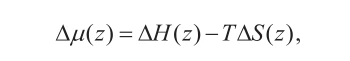(8)

where H is enthalpy and T is absolute temperature.

Generally, a force F(z) at position z in the channel is a gradient of potential energy. In one dimension it is:(9)

The overall force along the channel is given by integrating the force Fz(z) over the z axis from the entrance surface (in) to the output surface (out) of the channel:(10)

which gives the entropic driving force: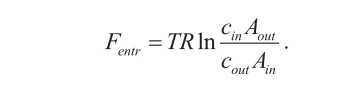(11)

Because the transport process is against the concentration gradient (cout/cin >1), the driving entropic force should fulfil the following conditions (we neglect the electric potential gradient, i.e., electrical field in the cell membrane):(12)

meaning that the channel has to be funnel shaped.

This is observed in the P-type ATPase proteins (Arguello et al., 2012). Therefore, we can model the channel by a conus (Figure 2) to more easily obtain a feeling for the relation between the geometry of the channel and the entropic driving force.Figure 2. Conical shape of channels (pores). The contribution of entropy to the active transport of ATPase is greater when the area of the exit surface of the cone available to the ion (Aout) is larger than the area of the entrance surface of the cone available to the ion (Ain).

Let rin be the radius of the input surface of the conus and rout be the radius of the output (exit) surface of the conus, while a is the radius of the transported ion. If we take into account the space available to the ion in the channel, then the force of entropy is: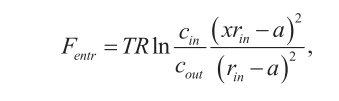(13)

where x = rout / rin.

To get a better feeling for the influence of geometrical conditions on the entropic force, we consider two limits. First when the channel is very large compared to the ion, i.e., when rin ➞ ∞ then the entropic force becomes: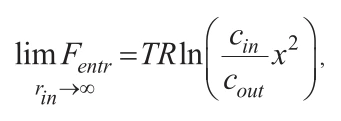(14)

which depends only on the ratio of input and output cross sections of the conus. As the channel becomes more funnel-shaped, the entropic force becomes stronger.

On the other hand, we consider a very narrow channel where the radius of the input cross section is very close to the radius of the ion. Then it follows that:(15)

That means that the entropic force is extremely strong for a narrow channel and is the dominant transfer mechanism of ions in active transport processes in such conditions.

The final result for entropic force (equation 13) is plotted in Figure 3 as a function of two variables, the ratios x = rout / rin and y = rin / a.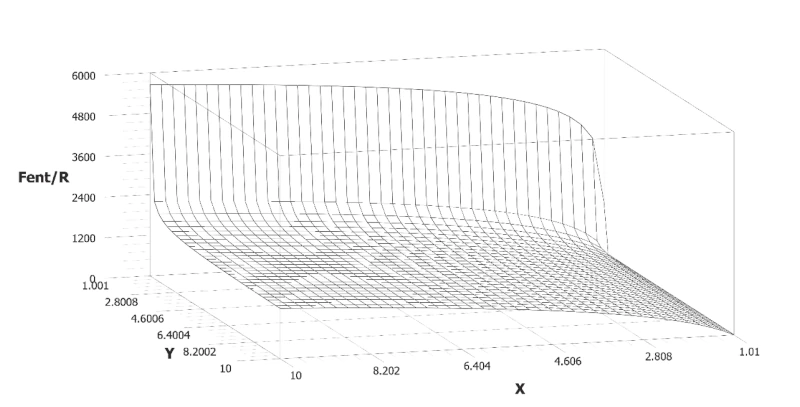Figure 3. Entropic force (Fent) expressed in terms of the gas constant R as a function of the ratios x = rout / rin and y = rin / a for the concentration ratio cin / cout = 1. It is evident that the force is zero for x = 1 (rout = rin), i.e., the square shape of the channel. Also, for values when y➞1 (rin➞a), i.e., when the channel is very narrow compared to the ion, the force becomes enormous, tending to infinity.

From the graph of the entropic force, we see that the force for x = 0 (the square-shaped channel) is zero and increases with increasing x values.
Also, as y values tend to zero the entropic force rises sharply. This is in accordance with our analyses of the entropic force in a narrow-channel limit.

In Table 1, we show the real values of geometry parameters (radii of entrance surfaces of channels and the ratio x = rout / rin) for Na+/K+-ATPase (Na+ only) (Takeuchi et al., 2009), Ca2+-ATPase (Olsen et al., 2007), Zn2+-ATPase (SITSEL et al., 2015), radii of Na+, Ca2+, Zn2+ ions (Marcus, 1988), calculated ratios y = rin / a and force due to entropy for Na+/K+-ATPase (Na+ only), Ca2+-ATPase, Zn2+-ATPase.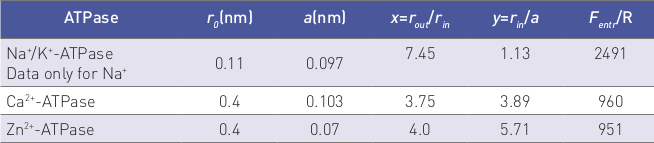Table 1. Values for radii of entrance surface of channels for Na+/K+-ATPase (Na+ only considered) (Takeuchi et al., 2009), Ca2+-ATPase (Olsen et al., 2007), Zn2+-ATPase (SITSEL et al., 2015), radii of ions taken (Marcus, 1988), ratios x = rout / rin and y = rin / a, force due to entropy on ions (calculated) are given.For Na+/K+-ATPase the force of entropy is much larger than for other ATPase due to narrowness of the channel and its very funnel shape.

## Discussion

We wanted to emphasise how the presented model of active transport of ions through the cell membrane solves three fundamental questions concerning the active transport of ions (substances): 1) How is a scalar quantity such as energy converted into a vector quantity such as the directional movement of ions?, 2) How can local energy release affect ion transport over the very large dimension of the channel length?, and 3) How does the released energy drive the ions, when is released far from the point of ion binding in the transport protein? The channel is much longer than its transverse dimensions (we marked them with the x and y axes). For this reason, local thermodynamic equilibrium is established very quickly in the x and y directions, meaning that entropy quickly reaches its maximum value, and has the same value at every point of that direction. That is why there is no entropy gradient in the x and y directions, and there is no transport of ions in those directions. The channel in the z direction extends over a much larger dimension, so the thermalisation process is significantly slowed. Therefore, an entropy gradient in the z direction is formed, transporting ions in that direction, and essentially making ion transport a quasi-one-dimensional motion. The model solves two additional questions. Namely, the release of energy leads to conformation at a large scale in the transport protein and the formation of a funnel-shaped channel.
The geometry of that channel produces an entropy gradient along the channel, which directly moves ions through the channel. In other words, energy moves ions far from the point of its release and the transport channel indirectly by the entropy gradient in the channel.

## Conclusion

Entropy is a key physical quantity in the transport of ions (substances) through the cell membrane. Although in the literature passive transport processes are defined as processes without energy consumption, we suggest that it could be enhanced by a statement that these are processes in which entropy increases. This would indicate that they are spontaneous (without external influence), irreversible and final processes, lasting for a very limited time, until entropy achieves its maximum value. In the presented model of active transport, entropy is the key quantity that transports ions through the cell membrane. Namely, the release of energy forms a funnel-shaped channel in which the cross-section of the channel exit is larger than the cross-section of the channel entrance. Such a geometry of the channel produces an entropy gradient that transports ions against the concentration gradient. The greater the ratio of the ion-available cross-section areas of the exit and entrance surfaces of the channel, the greater the force on the ions due to the entropy gradient. Our analysis of the entropic force in boundary conditions shows that for very wide channels, the force due to the entropy gradient is proportional to the ratio of the cross-sections of the exit and entrance surfaces of the channel, and the entropic force becomes enormous when the radius of the entrance surface of the channel approaches the radius of the ion (narrow channel).

References [… show]

## Entropija i njezino značenje u transportu iona kroz staničnu membranu

Dr. sc. Selim PAŠIĆ, mag. fizike, docent, Nato POPARA, mag. fizike, asistent, Veterinarski fakultet, Sveučilišta u Zagrebu, Hrvatska, Antea KLOBUČAR, dr. med. vet., Ministarstvo obrane Republike Hrvatske, Oružane snage Republike Hrvatske, Hrvatska ratna mornarica, Hrvatska; dr. sc. Denis CVITKOVIĆ, dr. med. vet., docent, dr. sc. Marinko VILIĆ, dr. med. vet., redoviti profesor, Veterinarski fakultet Sveučilišta u Zagrebu, Hrvatska

Svrha ovoga rada je predstaviti na jednostavan način koncept entropije i njezinu ulogu u transportu iona kroz staničnu membranu koristeći najnovije spoznaje iz biofizike. Na primjeru iz stvarnog života ukazujemo da procesi unutar nekoga sustava vode do porasta entropije, a prikazujemo da je porast entropije direktno povezan s ireverzibilnošću procesa i da određuje „vremensku strijelu“ – smjer protoka vremena. Na apstraktnom smo primjeru razjasnili pojam nereda sustava, koji se vrlo često koristi u definiciji entropije, povezujući ga s brojem mikrostanja koja ostvaruju određeno makroskopsko stanje. Razmatrali smo značenje entropije u transportnim procesima kroz staničnu membranu. Pokazali smo da su pasivni transportni procesi kroz staničnu membranu posljedica povećanja entropije u sustavu stanična membrana – transportna tvar. Detaljno smo prezentirali model aktivnoga transporta iona kroz staničnu membranu slijedeći Rubi i sur. (2017.). Sila koja transportira ione kroz kanal prouzročena je gradijentom entropije koji se prostire uzduž kanala, a posljedica je ljevkastoga oblika kanala. Sila entropije je proporcionalna omjeru ionu raspoloživih poprečnih presjeka izlazne i ulazne plohe kanala. To znači da kanal samo dostatno izraženoga ljevkastoga oblika može transportirati ione suprotno koncentracijskom gradijentu. Analizirali smo i konačni rezultat za entropijsku silu u graničnim uvjetima i izračunali da je za vrlo široki kanal sila proporcionalna omjeru površina ulazne i izlazne plohe, dok je za vrlo uzak kanal, čiji polumjer teži polumjeru iona, sila postaje izuzetno velika. Razumljivo smo objasnili kako prezentirani model rješava nekoliko otvorenih pitanja aktivnoga transporta tvari: kako se energija koja je skalarna veličina pretvara u usmjereno gibanje iona i što je vektorska veličina; kako energija transportira ione s obzirom da je mjesto oslobađanja energije daleko od točke vezivanja iona u transportnom proteinu; kako energija koje se oslobađa u vrlo uskom području može transportirati ione na velikoj skali.

Ključne riječi: entropija, aktivni transport, pasivni transport, ion, stanična membrana

Share...

#### Selim PAŠIĆ

Selim PAŠIĆ, BSc, PhD, Assistant Professor, Faculty of Veterinary Medicine, University of Zagreb, Croatia